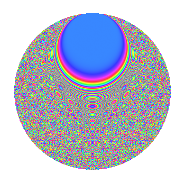# Properties

 Label 2015.2.erLevel 2015 Weight 2 Character orbit er Rep. character $$\chi_{2015}(151,\cdot)$$ Character field $$\Q(\zeta_{20})$$ Dimension 1216 Sturm bound 448

# Related objects

## Defining parameters

 Level: $$N$$ = $$2015 = 5 \cdot 13 \cdot 31$$ Weight: $$k$$ = $$2$$ Character orbit: $$[\chi]$$ = 2015.er (of order $$20$$ and degree $$8$$) Character conductor: $$\operatorname{cond}(\chi)$$ = $$403$$ Character field: $$\Q(\zeta_{20})$$ Sturm bound: $$448$$

## Dimensions

The following table gives the dimensions of various subspaces of $$M_{2}(2015, [\chi])$$.

Total New Old
Modular forms 1824 1216 608
Cusp forms 1760 1216 544
Eisenstein series 64 0 64

## Trace form

 $$1216q + 24q^{7} - 36q^{8} + 320q^{9} + O(q^{10})$$ $$1216q + 24q^{7} - 36q^{8} + 320q^{9} - 20q^{11} - 20q^{13} - 8q^{14} + 352q^{16} + 8q^{18} - 24q^{19} - 16q^{20} - 80q^{21} - 120q^{28} - 4q^{31} - 112q^{32} + 32q^{33} + 40q^{39} - 4q^{41} - 40q^{44} - 8q^{45} + 40q^{46} - 48q^{47} - 100q^{52} + 180q^{58} - 56q^{59} - 216q^{63} + 192q^{66} - 88q^{67} + 16q^{70} - 96q^{71} - 16q^{72} - 200q^{76} + 44q^{78} + 80q^{79} + 48q^{80} - 592q^{81} + 240q^{84} + 120q^{86} + 160q^{87} + 20q^{89} - 280q^{91} - 72q^{93} - 16q^{94} - 40q^{96} + 164q^{97} + 24q^{98} + O(q^{100})$$

## Decomposition of $$S_{2}^{\mathrm{new}}(2015, [\chi])$$ into irreducible Hecke orbits

The newforms in this space have not yet been added to the LMFDB.

## Decomposition of $$S_{2}^{\mathrm{old}}(2015, [\chi])$$ into lower level spaces

$$S_{2}^{\mathrm{old}}(2015, [\chi]) \cong$$ $$S_{2}^{\mathrm{new}}(403, [\chi])$$$$^{\oplus 2}$$